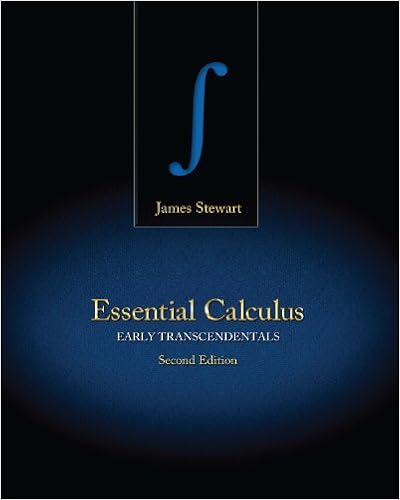How to Recognize/Sketch Vector Fields? 2 How to check if...

• Notes
• MasterDangerRabbit3360
• 25

This preview shows page 1 - 4 out of 25 pages.

We have textbook solutions for you!
The document you are viewing contains questions related to this textbook.The document you are viewing contains questions related to this textbook.
Chapter 13 / Exercise 5
Essential Calculus: Early Transcendentals
StewartExpert Verified
DEPARTMENT OF MATHEMATICAL SCIENCES CARNEGIE MELLON UNIVERSITY Math 21-259 Calculus in 3D Practice Problem from Chapter 13 What sections to expect from Chapter 13 in exam? Sections 13.1 - 13.8 . In particular, you should be able to answer the following questions. 1. How to Recognize/Sketch Vector Fields? 2. How to check if the given vector field is a gradient field or not? If a gradient field then how to find a potential function? 3. How to compute Line Integrals? (a) Directly. (b) Using Fundamental Theorem of Line integrals. (c) Using Green’s theorem (using double integral). (d) Stokes’ Theorem (using surface integral). 4. Application of Green’s Theorem in computing area of bounded regions. 5. Verify Green’s theorem. 6. How to compute Surface Area? 7. How to compute Surface Integrals? (a) Directly (b) Using Stokes’ theorem. 8. Verify Stokes Theorem. 1
We have textbook solutions for you!
The document you are viewing contains questions related to this textbook.The document you are viewing contains questions related to this textbook.
Chapter 13 / Exercise 5
Essential Calculus: Early Transcendentals
StewartExpert Verified
Practice Problems including Homework Problems 1. Evaluate the line integral R C ( y/x ) d s, C : x = t 4 , y = t 3 , 1 / 2 t 1 . (HW) 2. Evaluate the line integral R C xe y d x, C is the arc of the curve x = e y from (1, 0) to (e, 1). 3. Evaluate the line integral R C sin x d x + cos y d y, C consists of the top half of the circle x 2 + y 2 = 1 from (1, 0) to (-1, 0) and the line segments from (-1, 0) to (-2, 3).(HW) 4. Find the work done done by the force field F ( x, y ) = x sin y i + y j on a particle that moves along the parabola y = x 2 from (-1, 1) to (2, 4). (HW) 2
5. An object, acted on by various forces, moves along the parabola y = 3 x 2 from the origin to the point (1, 3). One of the forces acting on the object is F ( x, y ) = x 3 i + y j . Calculate the work done by F . 6. Determine the work done by the force F ( x, y, z ) = xy i + 2 j + 4 z k along the circular helix C : r ( u ) = cos u i + sin u j + u k , from u = 0 to u = 2 π. 3
•••